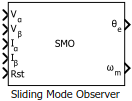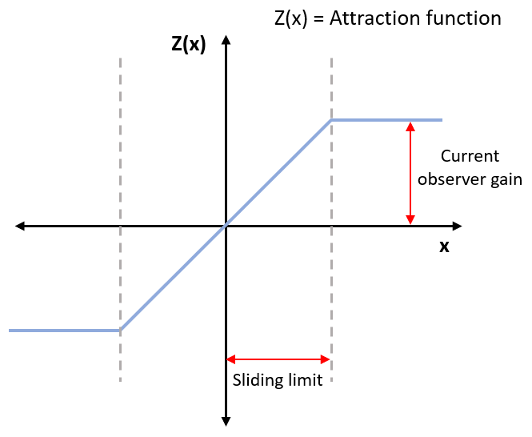# Sliding Mode Observer

Compute electrical position and mechanical speed of rotor

Since R2020a

•Libraries:
mcbpositiondecoderlib / Archive

## Description

The Sliding Mode Observer block computes the electrical position and mechanical speed of a PMSM by using the per unit voltage and current values along the α- and β-axes of the stationary αβ reference frame.

### Equations

These equations describe the computation of the electrical position and mechanical speed by the block.

`$\frac{d{i}_{\alpha \beta }}{dt}=\Phi {i}_{\alpha \beta }+\Gamma {V}_{\alpha \beta }-\Gamma {e}_{\alpha \beta }$`

`$\Phi =\left[\begin{array}{cc}-\frac{R}{L}& 0\\ 0& -\frac{R}{L}\end{array}\right]$`
`$\Gamma =\left[\begin{array}{cc}\frac{1}{L}& 0\\ 0& \frac{1}{L}\end{array}\right]$`

These equations describe the discrete-time sliding mode observer operation by using per-unit values:

`${\stackrel{^}{i}}_{\alpha \beta \left(k+1\right)P.U}=A{\stackrel{^}{i}}_{\alpha \beta \left(k\right)P.U}+\frac{{V}_{rated}}{{I}_{rated}}B\left({v}_{\alpha \beta \left(k\right)P.U}-{\vartheta }_{\alpha \beta \left(k\right)P.U}\right)$`
`${\vartheta }_{\alpha \beta \left(k+1\right)P.U}={\vartheta }_{\alpha \beta \left(k\right)P.U}+2\pi {f}_{0}×\left(Ζ\left({I}_{rated}\left({\stackrel{^}{i}}_{\alpha \beta \left(k\right)P.U}-{i}_{\alpha \beta \left(k\right)P.U}\right)\right)-{\vartheta }_{\alpha \beta \left(k\right)P.U}\right)$`
`$A={e}^{\Phi {T}_{s}}$`
`$B=\underset{0}{\overset{{T}_{s}}{\int }}{e}^{\Phi \tau }d\tau$`
`${f}_{0}=\frac{{F}_{0}}{{F}_{s}}$`
`${F}_{s}=\frac{1}{{T}_{s}}$`

where:

• are the stator back EMF and current for the α axis.

• are the stator back EMF and current for the β axis.

• ${v}_{\alpha },{v}_{\beta }$ are the stator supply voltages.

• $R$ is the stator resistance.

• $L$ is the stator inductance.

• $\psi$ is the flux linkage due to permanent magnet.

• ${\omega }_{e}$ is the electrical angular velocity.

• ${\theta }_{e}$ is the electrical position of the rotor.

• $t$ is the time.

• ${T}_{s}$ is the sampling period.

• $k$ is the sample count.

• ${V}_{rated}$ is the nominal voltage corresponding to 1 per-unit.

• ${I}_{rated}$ is the nominal current corresponding to 1 per-unit.

• $Z$ is the attraction function.• ${f}_{0}$ is the cut-off frequency of the filter in cycles per sample.

• ${F}_{0}$ is the cut-off frequency in cycles per second.

• ${F}_{s}$ is the sample frequency in samples per second.

• ${\vartheta }_{\alpha \beta \left(k\right)}$ is the estimated back EMF.

### Tuning

Use the Current observer gain and Sliding surface limit parameters to tune the block.

• To improve stability, increase the Sliding surface limit or reduce the Current observer gain.

• To reduce distortion, decrease the Current observer gain or increase the Sliding surface limit.

## Ports

### Input

expand all

Per-unit voltage component along the α-axis of the stationary αβ reference frame.

Data Types: `single` | `double` | `fixed point`

Per-unit voltage component along the β-axis of the stationary αβ reference frame.

Data Types: `single` | `double` | `fixed point`

Per-unit current component along the α-axis of the stationary αβ reference frame.

Data Types: `single` | `double` | `fixed point`

Per-unit current component along the β-axis of the stationary αβ reference frame.

Data Types: `single` | `double` | `fixed point`

The pulse (true value) that resets and restarts the processing of the block algorithm.

Data Types: `single` | `double` | `fixed point`

### Output

expand all

The estimated electrical position of the rotor.

Data Types: `single` | `double` | `fixed point`

The estimated mechanical speed of the rotor.

Data Types: `single` | `double` | `fixed point`

## Parameters

expand all

### Observer parameters

The attraction function gain.

The boundary layer limit of the attraction function's domain.

Unit of the position output.

Data type of the position output.

Unit of the speed output.

Data type of the speed output.

The fixed time interval (in seconds) between every two consecutive instances of block execution.

### Motor parameters

Stator phase winding resistance (in ohm).

Stator phase winding inductance (in Henry).

Maximum value of speed (in RPM) that the block can support. For a speed beyond this value, the block generates incorrect outputs.

Number of pole pairs available in the motor.

The maximum phase voltage applied to PMSM. For details, see Per-Unit System.

The maximum measurable current supplied to PMSM. For details, see Per-Unit System.

Note

The Sliding Mode Observer block may occasionally display the warning message `'Wrap on overflow detected`.`'`

 Y. Kung, N. V. Quynh, C. Huang and L. Huang, "Design and simulation of adaptive speed control for SMO-based sensorless PMSM drive," 2012 4th International Conference on Intelligent and Advanced Systems (ICIAS2012), Kuala Lumpur, 2012, pp. 439-444 (doi: 10.1109/ICIAS.2012.6306234)

 Zhang Yan and V. Utkin, "Sliding mode observers for electric machines-an overview," IEEE 2002 28th Annual Conference of the Industrial Electronics Society. IECON 02, Sevilla, 2002, pp. 1842-1847 vol.3. (doi: 10.1109/IECON.2002.1185251)

 T. Bernardes, V. F. Montagner, H. A. Gründling and H. Pinheiro, "Discrete-Time Sliding Mode Observer for Sensorless Vector Control of Permanent Magnet Synchronous Machine," in IEEE Transactions on Industrial Electronics, vol. 61, no. 4, pp. 1679-1691, April 2014 (doi: 10.1109/TIE.2013.2267700)

 Z. Guo and S. K. Panda, "Design of a sliding mode observer for sensorless control of SPMSM operating at medium and high speeds," 2015 IEEE Symposium on Sensorless Control for Electrical Drives (SLED), Sydney, NSW, 2015, pp. 1-6. (doi: 10.1109/SLED.2015.7339255)